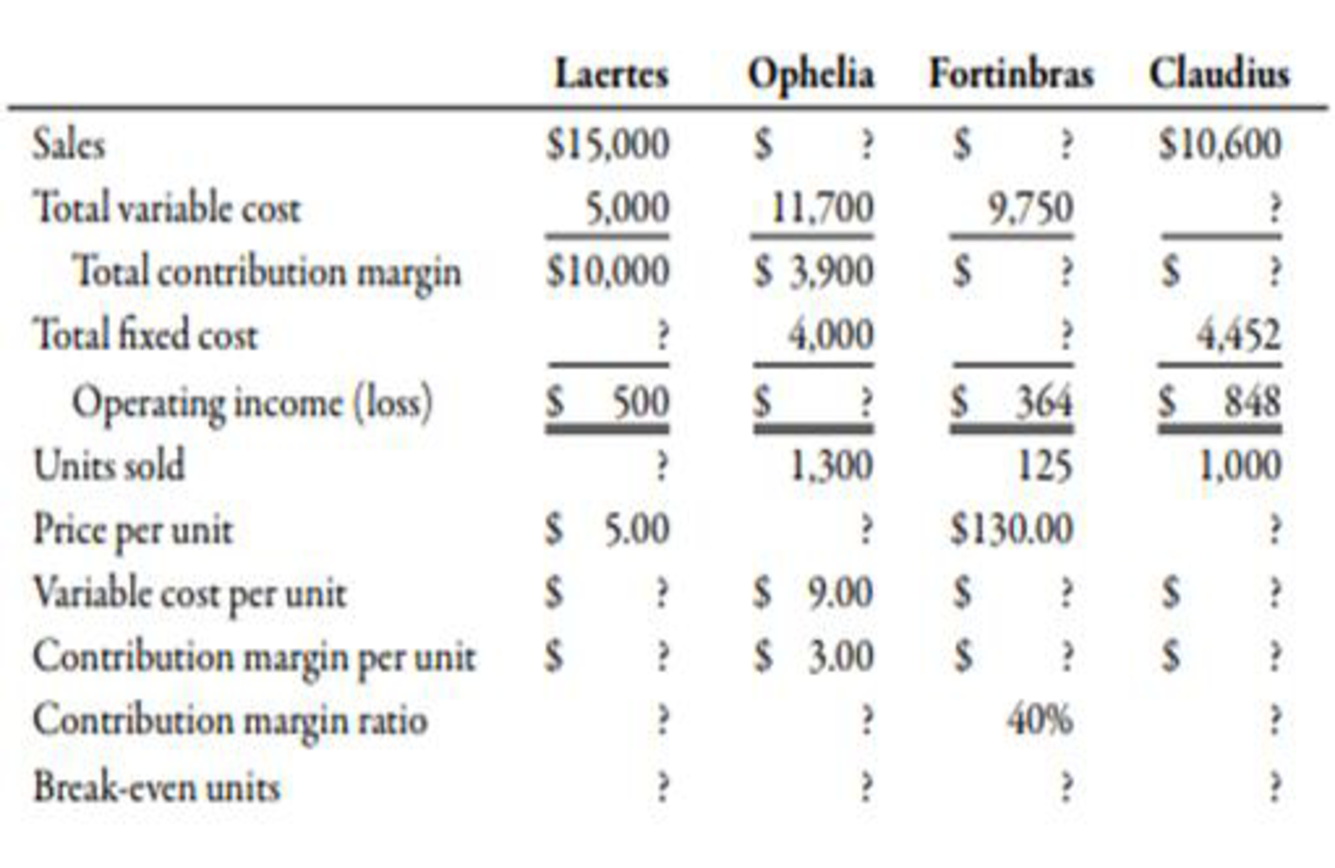Chapter 7, Problem 41E### Managerial Accounting: The Corners...

7th Edition
Maryanne M. Mowen + 2 others
ISBN: 9781337115773

#### Solutions

Chapter
Section### Managerial Accounting: The Corners...

7th Edition
Maryanne M. Mowen + 2 others
ISBN: 9781337115773
Textbook Problem
22 views

# Contribution Margin, Unit Amounts, Break-Even UnitsInformation on four independent companies follows. Calculate the correct amount for each question mark. (Note: Round unit dollar amounts and ratios to two decimal places; round break-even units to the nearest whole unit.)To determine

Compute the missing amount.

Explanation

Contribution Margin:

Contribution margin can be defined as the amount obtained after deducting the variable expense from sales revenue. It means the value of sales left after covering the variable expenses.

The following table represents the details of four companies with the help of calculations:

### Still sussing out bartleby?

Check out a sample textbook solution.

See a sample solution

#### The Solution to Your Study Problems

Bartleby provides explanations to thousands of textbook problems written by our experts, many with advanced degrees!

Get Started